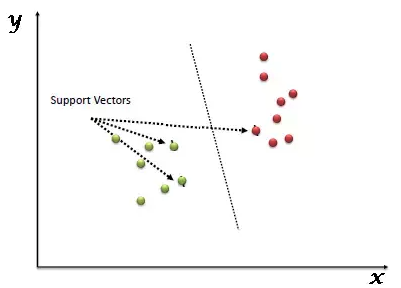# What is SVM?

The support vector machine (SVM) is a supervised machine learning model for two-group classification issues that takes into account classification techniques. The training data is represented as points in space that are split into groups with the assistance of a distinct separation that should be as large as feasible.

Support Vector Machine (SVM) is a supervised machine learning algorithm which can be used for both classification or regression challenges. However, it is mostly used in classification problems. In this algorithm, we plot each data item as a point in n-dimensional space (where n is number of features you have) with the value of each feature being the value of a particular coordinate. Then, we perform classification by finding the hyper-plane that differentiate the two classes very well (look at the below snapshot).Support Vectors are simply the co-ordinates of individual observation. Support Vector Machine is a frontier which best segregates the two classes (hyper-plane/ line).